Get instant live expert help with Excel or Google Sheets“My Excelchat expert helped me in less than 20 minutes, saving me what would have been 5 hours of work!”

#### Post your problem and you’ll get Expert help in seconds.

Your message must be at least 40 characters
Our professional Expert are available now. Your privacy is guaranteed.

# Rank with Criteria

Excel allows a user to get a rank based on the multiple criteria using COUNTIFS function. This step by step tutorial will assist all levels of Excel users in ranking data with several criteria.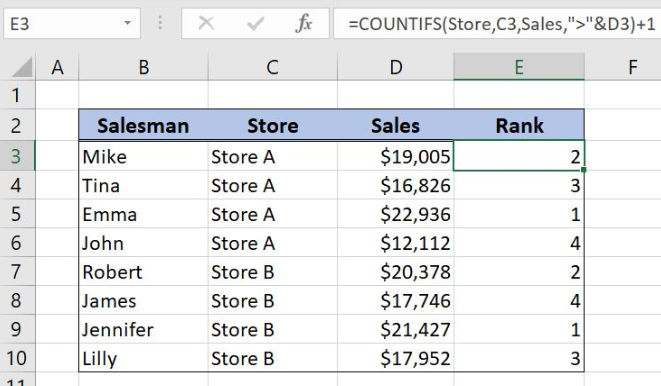Figure 1. Rank with criteria using COUNTIFS function

## Syntax of the COUNTIFS Formula

The generic formula for the COUNTIFS function is:

`=COUNTIFS(criteria_range1, criteria1, criteria_range2, criteria2, ... )`

The parameters of the COUNTIFS function are:

• criteria_range1, criteria_range2 – ranges where we want to apply our criteria
• criteria1, criteria2 – a criteria in criteria ranges which we want to count.

## Setting up Our Data to Rank with Criteria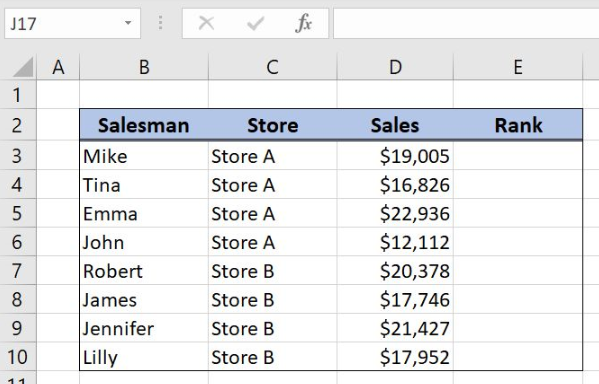Figure 2. Data that we will use in the example

In the data table, we have 4 columns: “Salesman” (column B), “Store” (column C), “Sales” (column D) and “Rank” (column E). The idea is to rank salesmen per store and sales values and to place the result in column E.

## Rank Salesmen per Store and Sales Values

In our example, we want to rank salesmen per store and sales values using COUNTIFS function. In order to make the formula more clear we will create two named ranges, Store for the cell range C3:C10 and Sales for the cell range D3:D10.

To create a named range we should follow the steps:

• Select the cell range that should be named
• Click on the name box in Excel
• Write the name for the cell range and press enter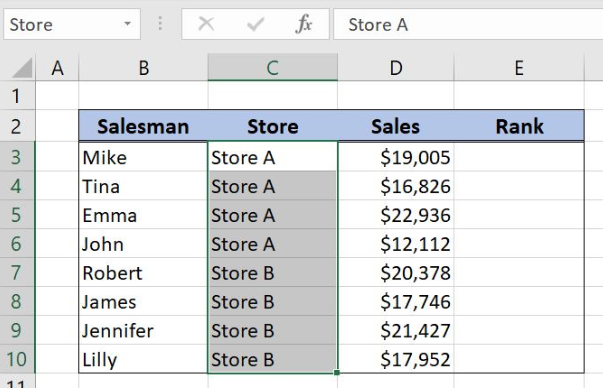Figure 3. Creating a named range Store for the COUNTIFS function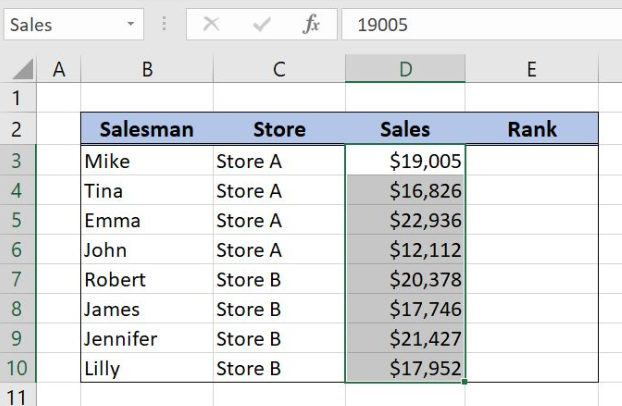Figure 4. Creating a named range Sales for the COUNTIFS function

The formula looks like:

`=COUNTIFS(Store,C3,Sales,">"&D3)+1`

The parameter criteria_range1 is named range Store and the criteria1 is in the cell C3. The parameter criteria_range2 is the named range Sales and the criteria2 is “>”&D3. On COUNTIFS formula result we should add number one.

To apply the function, we need to follow these steps:

• Select cell E3 and click on it
• Insert the formula: `=COUNTIFS(Store,C3,Sales,">"&D3)+1`
• Press enter
• Drag the formula down to the other cells in the column by clicking and dragging the little “+” icon at the bottom-right of the cell.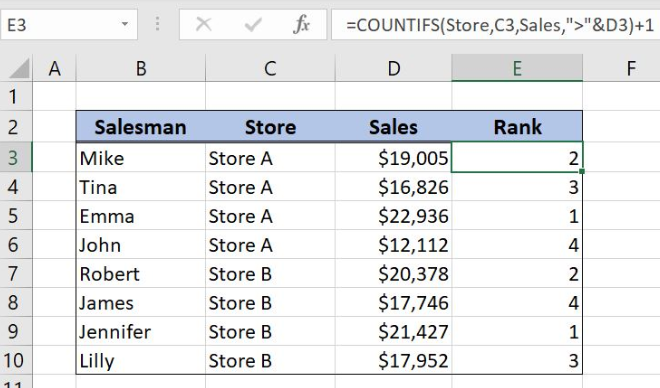Figure 5. Get the rank with criteria using COUNTIFS function

The first criteria in the COUNTIFS function is checking if the salesman belongs to the Store A or the Store B. The second condition is counting the number of the sales values that are greater than the value in the cell D3 for the Store A.

COUNTIFS formula output is the number 1 because there is one value for Store A that is greater than value \$19,005. To get the salesman rank we should add number 1 to the COUNTIFS formula result.

Most of the time, the problem you will need to solve will be more complex than a simple application of a formula or function. If you want to save hours of research and frustration, try our live Excelchat service! Our Excel Experts are available 24/7 to answer any Excel question you may have. We guarantee a connection within 30 seconds and a customized solution within 20 minutes.

### Did this post not answer your question? Get a solution from connecting with the expert.Another blog reader asked this question today on Excelchat:
Solution examplesi need a formula to find values in column f in worksheet 1 and count them.
Solved by B. A. in 20 minsI have a spreadsheet that contains a list of names/IDs and I want to generate a formula that inserts into a cell the number of times a name or ID appears on that list. Either insert a column into that spreadsheet or transfer that info into a column on a different spreadsheet. Either way would be fine.
Solved by O. L. in 18 minsi need a formula to count values that meet two conditions: one if a value is contained in a cell and another one is cells aren't empty
Solved by X. Q. in 20 minsGreetings, I am trying to find a formula in excel that will return the number of students attending courses. My data is on one sheet and my report will be on another sheet. The report is formated with the students list in a coulmn (far left), the courses are list in a row (top). My data is formatted with all columns, courses in column in "A" and students in column "B". Thanks
Solved by E. C. in 27 minsin range b2:o2, I am trying to write a formula to count if the values are equal to 1 thru 27.
Solved by E. L. in 52 mins## Subscribe to Excelchat.coAnother blog reader asked this question today on Excelchat: